# Electronics and Communication Engineering - Analog Electronics

21.

In a CE amplifier

 A. both ac and dc load lines have the same slope B. the ac load line has more slope than dc load line C. the ac load line has less slope than dc load line D. the two load lines may have slope more than the other

Explanation:

Since ac collector resistance is less than dc collector resistance, slope of ac load line is more than that of dc load line.

22.

The voltage gain of an amplifier without and with feedback are 100 and 20. The negative feedback is

 A. 4% B. 5% C. 20% D. 80%

Explanation: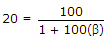or β = 0.04 or 4% .

23.

In the circuit shown in figure, RC = 10k, RE = 150Ω, β = 100, I = 1 mA. The value of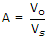, will be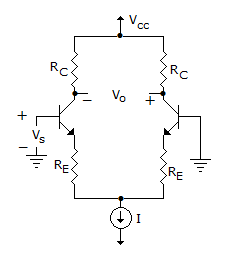A. 50 B. 100 C. 192 D. 400

Explanation:

circuit shown is Darlington Amplifier.

Gain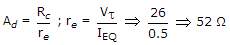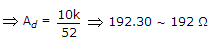.

24.

Calculate the conductivity of pure silicon at room temperature when the concentration of carriers is 1.6 x 1010 per cm3. Take μe = 1500 cm2/V-sec, μh = 500 cm2/V-sec at room temperature,

 A. 5.12 x 10-16 B. 5 x 10+16 C. 1016 D. 5 x 1020

Explanation:

σi = (μe + μn) . e . ni .

25.

A potential of 7 V is applied to a silicon diode. A resistance of 1 kΩ is also connected in series. If the diode is forward biased, the current in the circuit is

 A. 7 mA B. 6.3 mA C. 0.7 mA D. 0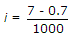A = 6.3 mA.